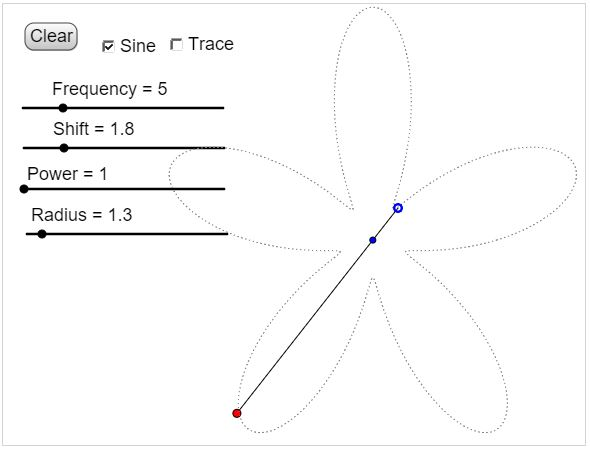# Equichordal Points: Just Do It

An interesting discussion was started on the geom.puzzles newsgroup with the following letter from Mary Krimmel.

I read: "A point inside a closed convex curve is called an equichordal point if all chords through that point have the same length. For example, the center of a circle is an equichordal point for that circle."

This is clear, but I can think of no other example. Are there others? If not, is there much purpose to making the definition?

Thank you, anyone.

Mary Krimmel

How would you answer Mary's question? Are there other examples?As it came out, Mary's question was related to a problem that has been around for more than 80 years and was solved as recently as 1997 by Marek Rychlik from University of Arizona.

A point P inside a closed convex curve C is called equichordal if every chord of C drawn through point P has the same length.Is there a curve with two equichordal points?

Rychlik solved the problem for a more general class of regions. He showed that no star - shaped region may contain more than one equichordal point.

This does not answer Mary's question, though. Are there convex curves other than the circle that have an equichordal point? (Which, from Rychlik's result, will have to be unique.) Surprisingly, the answer to that question is very simple: yes, of course. And there are many such curves. Moreover, it's easy to construct (or visualize, if you will) such curves. Imagine a fixed length rod constrained to rotate around a fixed point but let the rod be free to slide towards any of its ends. Just trace a curve with one of the ends eventually bringing it to the initial location of the other end. With a jerky mouse movements it is hard to get a region that looks remotely convex. It should become apparent though that such regions do exist.

17 February 2016, Created with GeoGebra

An example of a convex region can be obtained with a curve defined in polar coordinates, for example, by

r(t) = 2 + sin(t),

or, more generally, by $r(t) = f(t),\;$ where $f\;$ is any function that satisfies $f(t) + f(t + \pi ) = const.\;$ Starting with the sine function, we can get a four-parameter family of curves, of which some will be convex and some star - shaped. In the applet above, the curves are defined in the following manner:

$r(t) = Shift + Radius*[\sin(Frequency\cdot t)]^{Power},$

where both the frequency and the power are only allowed to be odd positive integers (why?).A region is star - shaped if there exists a point (not necessarily unique) that can be connected to any point on the boundary by a segment lying entirely in the interior of the region. All convex regions are star-shaped. In particular, triangles are star-shaped, as are quadrilaterals even if not convex. It's an interesting problem to find a non - star - shaped polygon with a minimum number of sides.